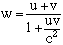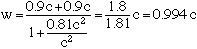Subject: Speed of light Name: Benny Lau Who is asking: Student Level: Secondary Question: I have a personal questions. In physics,if a person is running on a train, his relative speed on the ground will be the addition of the speed of train and the person. If u have a train inside another train and inside another train, so on...... can u reach the speed of light? It would need a very long distance. But if we make all the train travel in circles. Then we don't need that much distance. So can we achieve the speed of Light? Similar situation would be a plane faster than speed of sound, launch a missle, and that missle keep on launching more missle. Would that achieve the speed of light as well. Of course Air Resistace would be a great problem, but if we do it in space, can we achieve the speed of light. Thank you for answering my question. Hi Benny, Your statement that "his relative speed on the ground will be the addition of the speed of train and the person" is true in Newtonian mechanics where objects are travelling at speeds that are much below the speed of light. In the theory of relativity however adding velocities is not as straightforward. If u and v are the speeds of the two trains and c is the speed of light in the same units then w, the speed of the inside train relative to the ground, is given byIf u and v are small in relation to c, as would be true with the second train inside the first, then uv/c2 would be very close to zero and hence w would be very close to u + v. If however u = v = 0.9 c thenstill less than c. Cheers, Robert and Harley Go to Math Central